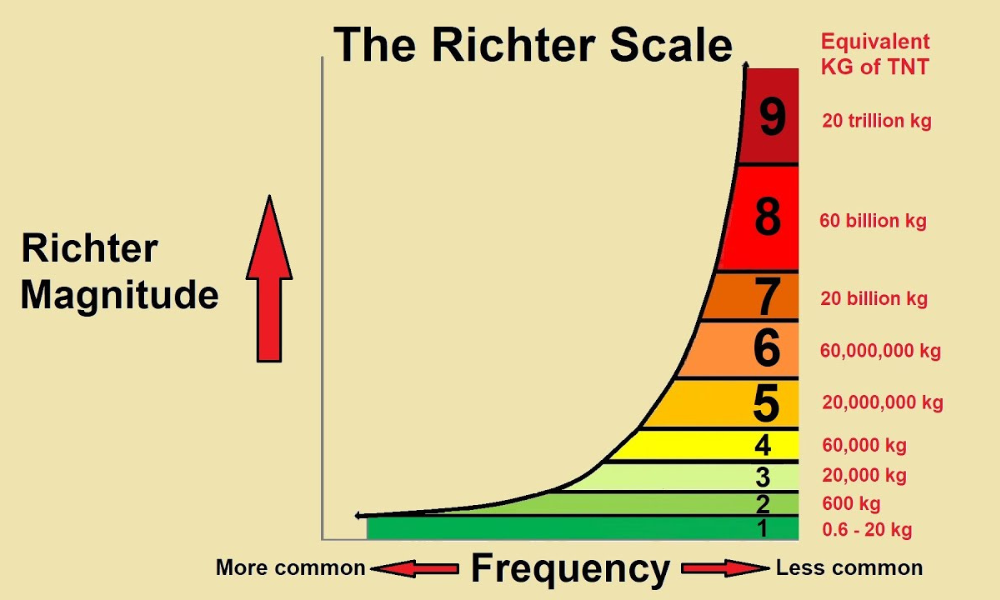# Understanding the Richter Scale: Measuring Earthquake Magnitude

Earthquakes are natural disasters that can strike with little to no warning, causing widespread destruction and loss of life.

To understand and effectively respond to these seismic events, scientists rely on various tools and scales to measure their magnitude and assess their impact.

One such scale is the Richter Scale, which has played a crucial role in earthquake science for many decades.

In this blog, we'll delve into the Richter Scale and explore how earthquake magnitude is measured.

 Are you a Tax Lawyer in USA?   👉Transform Your Brand: Click for Metamorphosis👈

## The Richter Scale: A Brief Overview

The Richter Scale, also known as the Richter magnitude scale, was developed in 1935 by American seismologists Charles F. Richter and Beno Gutenberg.

This scale quantifies the energy released by an earthquake and assigns it a numerical value called the Richter magnitude, commonly referred to as "magnitude."

The Richter Scale is logarithmic, meaning that each whole number increase on the scale represents a tenfold increase in amplitude of the seismic waves and approximately 31.6 times more energy release.

## How is Earthquake Magnitude Measured?

1. ### Seismographs: The Foundation of Measurement

The primary tool for measuring earthquake magnitude is the seismograph, an instrument that detects and records ground motion caused by seismic waves.

When an earthquake occurs, it generates seismic waves that travel through the Earth's crust and are detected by seismographs.

These instruments consist of a mass suspended on a spring, with a pen attached to the mass and a rotating drum covered in paper beneath it.

As the ground shakes, the drum moves, causing the pen to draw a wavy line called a seismogram.

1. ### Calculating Amplitude

To determine the magnitude of an earthquake, scientists examine the amplitude of the seismic waves recorded on the seismogram.

The amplitude is the height of the wavy lines, and it directly correlates with the energy released during the quake.

Larger amplitudes indicate more powerful earthquakes.

1. ### Distance Matters

Another critical factor in calculating earthquake magnitude is the distance between the earthquake's epicenter (the point on the Earth's surface directly above the seismic source) and the seismograph station recording the waves.

This distance helps account for the spread of energy as seismic waves travel from the source to the recording station.

The greater the distance, the smaller the amplitude of the waves will appear on the seismogram.

1. ### Richter's Formula

Charles F. Richter developed a formula to calculate earthquake magnitude using the amplitude and distance data collected from seismograms.

The formula looks like this:

• Magnitude = log10(A / A0) + 3 * log10(D / D0)

In this formula:

• Magnitude is the Richter magnitude of the earthquake.
• A is the amplitude of the seismic waves recorded on the seismogram.
• A0 is a reference amplitude (a constant value).
• D is the distance between the epicenter and the seismograph station.
• D0 is a reference distance (a constant value).

By plugging these values into the formula, scientists can calculate the Richter magnitude of an earthquake accurately.## Conclusion

The Richter Scale has been an invaluable tool in earthquake science, helping researchers assess the magnitude and energy release of seismic events.

Understanding how this scale works, along with the use of seismographs and mathematical formulas, allows scientists and emergency responders to better prepare for and respond to earthquakes, potentially saving lives and reducing the impact of these natural disasters.

While other magnitude scales have been developed over the years, the Richter Scale remains a fundamental and widely recognized method for measuring earthquake magnitude.